# Writing your own block with discrete states (MATLAB S-Function)

A few weeks ago, I noticed the following question on MATLAB Answers by K E:

What are continuous and discrete states in Simulink?

In my opinion, the best way to understand how states work in Simulink is by implementing an S-Function. So this week we will see how to create a MATLAB S-Function with discrete states.

If you are not familiar with MATLAB S-Functions, I recommend looking at my previous post introducing the basics of MATLAB S-Functions

What is a state?

Before starting to implement our S-Function, let's see the answer my friend Kaustubha Govind provided to the above question:

In my own words, a crude word to use in place of "state" is "memory". A state adds memory to a system in such a way that the output at a given time depends not only on its current input, but also on its previous inputs. There is a more formal explanation about states here.

Discrete states can be thought purely as internal memory - for example a Unit Delay block has one discrete state...

Let's stop here and see how to implement a Unit Delay in a MATLAB S-Function.

Setup

In the setup function, we define one parameter, one input port and one output port.

Direct Feedthrough?

"Does this block have Direct Feedthrough?" This is the question Simulink needs to know to determine the sorting order for all the blocks in the model. Direct feedthrough means that the Outputs function uses the input variable in its calculation. Since we are not planning to use the input value in the Outputs function to calculate the output value, we can set the DirectFeedthrough property of the input port to false.

Finally, we need to register 4 methods: PostPropagationSetup, InitializeConditions, Outputs and Update.

Here is how this looks: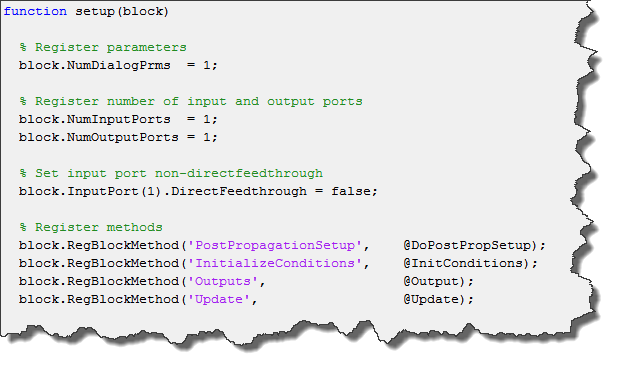Post Propagation Setup

In terms of S-Function, the discrete state is stored in what we call a work vector, the Dwork. The properties of the work vector are defined in the DoPostPropSetup callback method.

In our case, we need one Dwork vector, of dimension 1, and we want to mark it as a discrete state: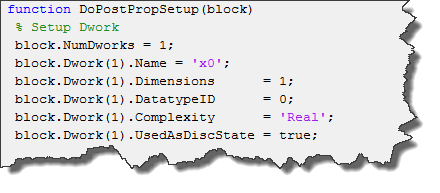Setting the UsedAsDiscState to true makes the states visible to the Simulink engine. That way it will be included if we save the model states and will be seen by Simulink.BlockDiagram.getInitialState. If you prefer to hide the internal state of your block, you can set UsedAsDiscState to false.

Initial Conditions

When the simulation starts, you can set the initial value of the state. Here we do it using a dialog parameter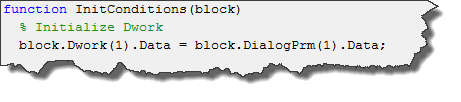Outputs

Now the simulation loop can start. First, the Outputs method of the block is called. We read the value stored in the work vector and assign it to the output port: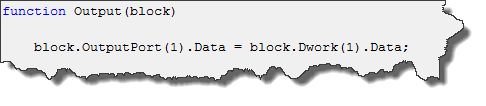Update

After the Outputs method is completed, we grab the input and store it in the work vector. This is done in the Update method: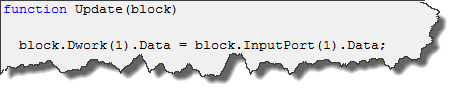The next time the Outputs method runs, this value will be available.

Conclusion

It is important to realize here that Simulink does nothing to the discrete states. The solver only stores the values to be used in the next step.

Next week, we will follow a similar process with continuous states.